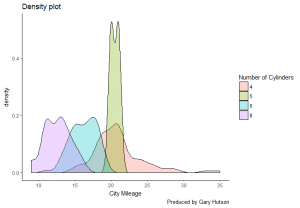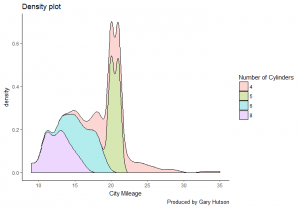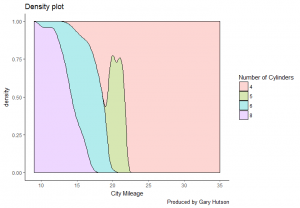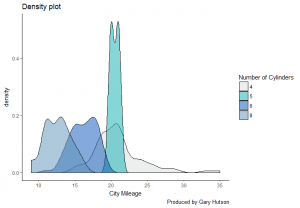[This article was first published on R Blogs – Hutsons-hacks, and kindly contributed to R-bloggers]. (You can report issue about the content on this page here)
Want to share your content on R-bloggers? click here if you have a blog, or here if you don't.

## Violin Plots

This chart is a combination of a Box Plot and a Density Plo that is rotated and placed on each side, to show the distribution shape of the data. The thick black bar in the centre represents the interquartile range, the thin black line extended from it represents the 95% confidence intervals, and the white dot is the median. The graphic hereunder illustrates how these should be interpreted:With that background, we will use the geom_violin() geometry with the same dataset as we worked with in the histogram blog entitled: Box Plots in ggplot2.

### Creating the Violin Plot in R

The code below show how to create a simple violin plot in R:

```library(ggplot2)
theme_set(theme_bw())
# plot
plot <- ggplot(mpg, aes(class, cty))
plot + geom_violin(fill="blue") +
labs(title="Violin plot",
caption="Produced by Gary Hutson",
x="Class of Vehicle",y="Mileage") +
geom_jitter(height = 0, width = 0.1, colour="black")
```

This uses the ggplot library and sets a theme for the chart. Then the plot is created from the mpg dataset we worked with in the Box Plot section. Once the plot placeholder has been used, we then add the geom_violin() layer and make the area of the violin plot blue, you could also use an aes layer and set the aesthetics equal to a factor within the dataset. The other part is the label code and at the very end I add another geometry to jitter the points on the violin, indicating that the points should be black and forcing a slight offset (width = 0.1) to each of the points. This enables the recipient of the diagram to observe how the points are distributed. Running the code will give you the result, as below:Personally, I am not a big fan of this visualisation technique, but this serves as a demonstration to show how they can be created in R. The next plotting type, in this blog, will be a density plot.

## Density Plots

A Density Plot visualises the distribution of data over a continuous interval or time period. This chart is a variation of a Histogram that uses kernel smoothing to plot values, allowing for smoother distributions by smoothing out the noise. The peaks of a Density Plot help display where values are concentrated over the interval. The advantage of these plots are that they are better at determining the shape of a distribution, due to the fact that they do not use bins. With that fresh in memory I will show you how to create these in R.

### Creating the Density Plot in R

The code below changes the density plot:

```library(ggplot2)
theme_set(theme_classic())
plot <- ggplot(mpg, aes(cty))
plot + geom_density(aes(fill=factor(cyl)), alpha=0.3) +
labs(title="Density plot",
caption="Produced by Gary Hutson",
x="City Mileage",
fill="Number of Cylinders")
```

We use the same setup as before and after we have set the initial plot placeholder we then add the plot placeholder to the geom_density() geometry. In this geometry I specify that I want the fill of the densities to be equal to a factor of the number of cylinder each vehicle has and then I change the alpha value to change the transparency of the densities. Then, add labels as per many of the previous examples. The result is as below:You can change the type of chart by adding the position parameter to the geometry layer for densities:

```plot + geom_density(aes(fill=factor(cyl)), alpha=0.3, position="stack")
```

This stacks the densities and the ouput looks as below:The position argument can also be changed to fill:

```plot + geom_density(aes(fill=factor(cyl)), alpha=0.3, position="fill")
```

Resultant output:One last tip before I depart. To change the colours of any plot you can create a combination of palette colours. A custom colour palette can be specified using the functions :

• scale_fill_manual() for box plot, bar plot, violin plot, etc.
• scale_color_manual() for lines and points

The example below shows how to use hexadecimal colours for the 4 factor values for cylinders, obviously the number of manual colours must match the number of factors:

```library(ggplot2)
theme_set(theme_classic())

#Specify custom palette
plot <- ggplot(mpg, aes(cty))
plot + geom_density(aes(fill=factor(cyl)), alpha=0.5) +
labs(title="Density plot",
caption="Produced by Gary Hutson",
x="City Mileage",
fill="Number of Cylinders") +
#I force the scale to conform to certain hexadecimal colour codes
scale_fill_manual(values=c("#dbdfe0", "#0faeb1",
"#0954b7", "#5c93b8"))
#scale_fill_brewer(palette="Dark2")
```

The scale_fill_manual values have been set, these could be rgb colours or base R colours. The output has now been customised somewhat:An alternative would be to use the scale_fill_brewer() command and use a palette to fill the densities.

##### This post appears on R-Bloggers - please check out all the other cool blogs featured on this site.

To leave a comment for the author, please follow the link and comment on their blog: R Blogs – Hutsons-hacks.

R-bloggers.com offers daily e-mail updates about R news and tutorials about learning R and many other topics. Click here if you're looking to post or find an R/data-science job.
Want to share your content on R-bloggers? click here if you have a blog, or here if you don't.

# Never miss an update! Subscribe to R-bloggers to receive e-mails with the latest R posts.(You will not see this message again.)

Click here to close (This popup will not appear again)